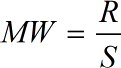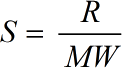Request a Tool

Specific Gas Constant Calculator

Want to calculate gas constant? So, just put values in input box to use this Gas constant calculator.

Molecular Weight
0

Formula• MW = Molecular Weight
• S = Specific Gas Constant
• R = Universal Gas Constant = 8314 J/kmol-K

Defination / Uses

The Specific Gas Constant refers to the constant of gas which is equal to 8.314 joules per Kelvin or 1.985 calories per degree Celsius. This figure is the constant of proportionality [R] in the equation Pressure * Volume = n (number of moles) * (R) * Temperature, relating the pressure and volume of a quantity of gas to the absolute temperature. The specific gas constant is denoted by the symbol 'R specific'.

The Specific Gas Constant is defined as the gas constant divided by the molecular weight of a gas. This online Specific Gas Constant Calculator is useful in calculating the gas constant.

Output

Specific Gas Constant
0

Formula• S = Specific Gas Constant
• MW = Molecular Weight
• R = Universal Gas Constant = 8314 J/kmol-K

Defination / Uses

The Specific Gas Constant refers to the constant of gas which is equal to 8.314 joules per Kelvin or 1.985 calories per degree Celsius. This figure is the constant of proportionality [R] in the equation Pressure x Volume = n (number of moles) x (R) x Temperature, relating the pressure and volume of a quantity of gas to the absolute temperature. The specific gas constant is denoted by the symbol 'R specific'.

The Specific Gas Constant is defined as the gas constant divided by the molecular weight of a gas. This online Specific Gas Constant Calculator is useful in calculating the gas constant.

How to use this calcultor?

There are only two basic steps.

• First select the option from dropdown. What you need to calculate.
• Then add the otherone value in its corresponding field.